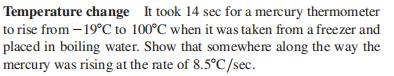### Still have math questions?Temperature change It took $$14 sec$$ for a mercury thermometer to rise from $$- 19 ^ { \circ } C$$ to $$100 ^ { \circ } C$$ when it was taken from a freezer and placed in boiling water. Show that somewhere along the way the mercury was rising at the rate of $$8.5 ^ { \circ } C / sec$$ .
$$\left. \begin{array} { l } { \text { If } T ( t ) \text { is the temperature of the thermometer at time } t \text { , then } } \\ { T ( 0 ) = - 19 ^ { \circ } C \text { and } T ( 14 ) = 100 ^ { \circ } C \text { . From the Mean Value } } \\ { \text { Theorem, there exists a } 0 < t _ { 0 } < 14 \text { such that } } \\ { \frac { T ( 14 ) - T ( 0 ) } { 14 - 0 } = 8.5 ^ { \circ } C / sec = T ^ { \prime } ( t _ { 0 } ) \text { , the rate at which the } } \\ { \text { temperature was changing at } t = t _ { 0 } \text { as measured by the rising } } \\ { \text { mercury on the thermometer. } } \end{array} \right.$$